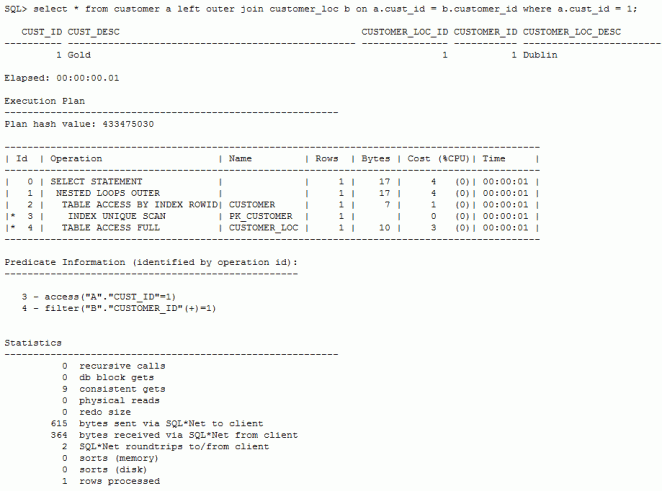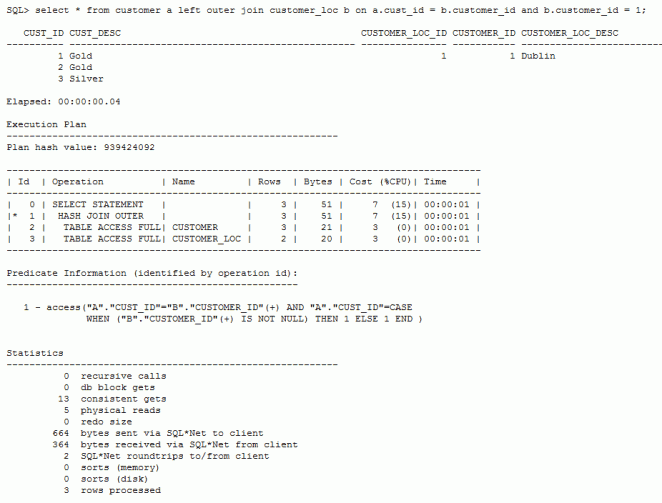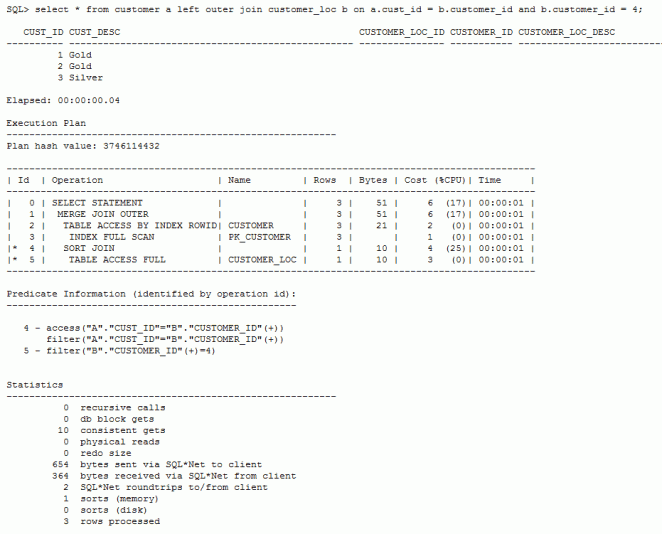## Wednesday, 31 December 2014

### Removing Special Characters from a string field in Oracle

Syntax:

Select Regexp_Replace( Column_name ,'[^[:alnum:]'' '']', Null)  FROM table_name ;

Example:

Select Regexp_Replace( '@#@#@##Customer_Description' ,'[^[:alnum:]'' '']', Null)  FROM dual

Explanation

The [[:alnum:]] character class represents alphabetic and numeric characters, and it is same as using [a-zA-Z0-9] in regular expression.

## Sunday, 28 December 2014

### Query to get count of all tables in a database/schema

Syntax:

select object_name table_name,to_number(
extractvalue(dbms_xmlgen.getxmltype('select count(*) cnt from ' || object_NAME),'/ROWSET/ROW/CNT')) NUM_ROWS
from all_objects where object_type='TABLE' and owner='&OWNERNAME' order by 1

## Wednesday, 24 December 2014

### Query to get count of all tables in a Oracle Database for Specific User

Query :

select table_name
, to_number
( extractvalue
( dbms_xmlgen.getxmltype('select count(*) c from ' || table_name)
, '/ROWSET/ROW/C'
)
) cnt
from user_tables
order by table_name

Example :
If you have logged in as user 'A' then the above query will display all the tables and count information available for User 'A'.

## What is the approximate size of a data warehouse?

You can estimate the approximate size of a data warehouse made up of only fact and dimension tables by estimating the approximate size of the fact tables and ignoring the sizes of the dimension tables.

To estimate the size of the fact table in bytes, multiply the size of a row by the number of rows in the fact table.

A more exact estimate would include the data types, indexes, page sizes, etc. An estimate of the number of rows in the fact table is obtained by multiplying the number of transactions per hour by the number of hours in a typical work day and then multiplying the result by the number of days in a year and finally multiply this result by the number of years of transactions involved. Divide this result by 1024 to convert to kilobytes and by 1024 again to convert to megabytes.

E.g. A data warehouse will store facts about the help provided by a company’s product support representatives. The fact table is made of up of a composite key of 7 indexes (int data type) including the primary key. The fact table also contains 1 measure of time (datetime data type) and another measure of duration (int data type). 2000 product incidents are recorded each hour in a relational database.

A typical work day is 8 hours and support is provided for every day in the year. What will be approximate size of this data warehouse in 5 years?

• First calculate the approximate size of a row in bytes (int data type = 4 bytes, datetime data type = 8 bytes):

• Size of a row = size of all composite indexes (add the size of all indexes) + size of all measures (add the size of all measures).

• Size of a row (bytes) = (4 * 7) + (8 + 4).

• Size of a row (bytes) = 40 bytes.

• Number of rows in fact table = (number of transactions per hour) * (8 hours) * (365 days in a year).

• Number of rows in fact table = (2000 product incidents per hour) * (8 Hours ) * (365 days in a year).

• Number of rows in fact table = 2000 * 8 * 365

• Number of rows in fact table = 5840000

• Size of fact table (1 year) = (Number of rows in fact table) * (Size of a row)

• Size of fact table (bytes per year) = 5840000 * 40 Size of fact table (bytes per year) = 233600000.

• Size of fact table (megabytes per year) = 233600000 / (1024*1024)

• Size of fact table (in megabytes for 5 years) = (23360000 * 5) / (1024 *1024)

• Size of fact table (megabytes) = 1113.89 MB

• Size of fact table (gigabytes) = 1113.89 / 1024

• Size of fact table (gigabytes) = 1.089 GB

### Arithmetic Calculation in Shell Scripts

To do arithmetic calculation we can use either expr or bc.

EXPR Syntax:

`expr \$A + \$B` (Space is Mandatory)

BC Syntax:

\$A+\$B|bc

### OBIA 11.1.1.7.1 / 11.1.1.8.1 Custom BIACM Offerings for Load Plan / Custom Fact Group

BIACM Config
Step 1: Creation of Custom Offering
Step 2: Creation of Source for the new offering
Step 3: Creation of Custom Functional Area
Step 4: Creation of relation between Offering and Functional Area
Step 5:  Creation of fact group (Repeat for each Fact Group)
Step 6: Creation of Func Area to Fact Group Relation (Repeat for each Fact Group)
Linking BIACM Fact Groups to ODI Objects
Fact Model / Dimension Model
Load Plan Steps (provided only for SILs, but SDE steps will be the same)
Dev Components
Load Plan System Component (Required for a New Fact Group or Dim Group)
Generate the Custom Load Plan in BIACM

## Friday, 12 December 2014

### BEWARE OF THE PREDICATE IN THE OUTER JOIN

Today we will have a look what happens when we place a filter condition into an outer join. We will also have a look at how this compares to placing the filter condition into the WHERE clause. Finally we’ll have a look where this could be useful.

Let’s first create some sample data.

 `1` `create` `table` `customer (`
 `2` `cust_id number,`
 `3` `cust_desc varchar2(50)`
 `4` `);`
 `5`
 `6` `ALTER` `TABLE` `customer`
 `7` `add` `CONSTRAINT` `pk_customer ``PRIMARY` `KEY` `(cust_id);`
 `8`
 `9` `create` `table` `customer_loc (`
 `10` `customer_loc_id NUMBER,`
 `11` `customer_id NUMBER,`
 `12` `customer_loc_desc VARCHAR2(50)`
 `13` `);`
 `14`
 `15` `ALTER` `TABLE` `customer_loc`
 `16` `add` `CONSTRAINT` `pk_customer_loc ``PRIMARY` `KEY` `(customer_loc_id);`
 `17`
 `18` `insert` `into` `customer ``values` `(1,``'Gold'``);`
 `19` `insert` `into` `customer ``values` `(2,``'Gold'``);`
 `20` `insert` `into` `customer ``values` `(3,``'Silver'``);`
 `21`
 `22` `insert` `into` `customer_loc ``values` `(1,1,``'Dublin'``);`
 `23` `insert` `into` `customer_loc ``values` `(2,2,``'Paris'``);`
 `24` `insert` `into` `customer_loc ``values` `(3,4,``'Berlin'``);`
 `25`
 `26` `commit``;`
 `27`
 `28` `analyze ``table` `customer compute ``statistics``;`
 `29` `analyze ``table` `customer_loc compute ``statistics``;`

Let’s run our first outer join query where we put the filter condition into the WHERE clause. All is as expected. The query just returns customer_id 1.Now let’s run the same query, but we put the filter condition into the join. This may be different from what you may have expected. What happens is that the query returns all of the rows in the customer table and those rows of table customer_loc where the join condition is met, i.e. those rows where customer_id = 1.Let’s verify what we have just seen with another query. This time we will put customer_id = 4 into the Join condition. There are no rows in table customer_loc that match this. As expected the query returns all rows for table customer but now rows for table customer_locWhat could this be useful for? One use case would be some limited form of data densification whereby you need to return all of the tables in one table but only a subset of rows in another table. Typically this can only be done using some sort of subselect. An example:

The query below is run in the SH schema and returns all of the customers, but only those sales transactions that are larger than 1000 in sales_amount.

 `1` `select` `a.cust_id,amount_sold`
 `2` `from` `customers a`
 `3` `left` `outer` `join` `sales b ``on` `a.cust_id = b.cust_id ``and` `amount_sold > 1000;`